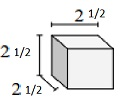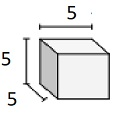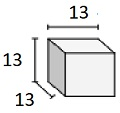# Volume of a Solid made of Cubes with Unit Fraction Edge Lengths

#### Le Guide Définitif du Développement Web HTML.

76 Lectures 3.5 hours

#### SAP ERP : Gestion magasin WM (débutant et avancé)

66 Lectures 4.5 hours

#### Initiation SAP ERP débutant - Les Bases

12 Lectures 1 hours

Here we find volume of solids made of cubes with unit fraction edge lengths. Consider for example a solid of dimensions 3 in × 3 in × 3 made of small cubes with $\frac{1}{2}$ inch edge lengths.

In that case the solid is made up of 6 × 6 × 6 small cubes of $\frac{1}{2}$ inch edge lengths. So the volume of the solid in this case would be

Volume = l w h = $6 \times \frac{1}{2} \times 6 \times \frac{1}{2} \times 6 \times \frac{1}{2}$

= 3 × 3 × 3 = 27 cubic inchesFormula for the volume of solid made of cubes with unit fractional edge lengths

Assuming the solid to be a cube of edge a units

b = number of cubes with unit fractional edge length along each edge

k = unit fractional edge length

Volume of solid = b × k × b × k × b × k cubic units

Find the volume of following solid of cubes with unit fraction edge lengths. Each prisms unit is measured in cm (not to scale)### Solution

Step 1:

Solid of cubes with unit fraction edge lengths of $\frac{1}{2}$ cmStep 2:

Volume V = l w h = $2 \frac{1}{2} \times 2 \frac{1}{2} \times 2 \frac{1}{2}$

= $5 \times \frac{1}{2} \times 5 \times \frac{1}{2} \times 5 \times \frac{1}{2}$

= $15 \frac{5}{8}$ cu cm

Find the volume of following solid of cubes with unit fraction edge lengths. Each prisms unit is measured in cm (not to scale)### Solution

Step 1:

Solid of cubes with unit fraction edge lengths of $\frac{1}{3}$ cmStep 2:

Volume V = l w h = $4 \frac{1}{3} \times 4 \frac{1}{3} \times 4 \frac{1}{3}$

= $13 \times \frac{1}{3} \times 13 \times \frac{1}{3} \times 13 \times \frac{1}{3}$

= $81 \frac{10}{27}$ cu cm